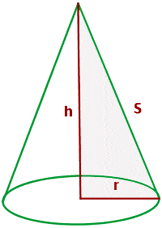Are you looking for formulas for the calculation of the volume of different polyhedrons? Then you came to the right place! In this resource, we made a list of formulas for volume for different polyhedrons. A handful of formulas will help you increase your productivity.

### TetrahedronThe volume of a tetrahedron is the product of the cube of the edge of the tetrahedron and the ratio of the square root of 2 and twelve and the cube of the edge of the tetrahedron.

OctahedronThe volume of an octahedron is the product of the cube of the edge of the octahedron and the ratio of the square root of two and 3.

### IcosahedronorThe above formula is for an icosahedron that contains 20 faces. The variable "a" denotes the edge of a icosahedron.

### DodecahedronThe above formula is the formula for volume of a dodecahedron that contains twelve faces. All you need is the length of an edge of dodecahedron to find the volume.

### CubeThe volume of a cube is equal to the cube of an edge of a cube.

CuboidThe volume of a cuboid is the product of all three dimensions of a cuboid.

PrismThe volume of the prism is equal to the product of the base area and height between the top and the bottom of the base.

### PyramidTo find the volume of a pyramid, you need the base area and the height from the base to the top point. Once you have the information, multiply the base area with the height and then divide the total value by 3.

### Truncated PyramidA = Area of the larger base

A' = Area of the smaller baseTo find the area of a truncated pyramid, you need the height between both bases as well as the area of both bases. Use the above formula to calculate the volume of a truncated pyramid.

### CylinderThe volume of the cylinder is the product of two pi, the square of the radius, and the height of the cylinder.

ConeTo find the volume of a cone, multiply the value of pi with the square of the base radius of the cone and the height of the cone. Then divide the total value by three and the final value will be the volume of a cone.

Truncated ConeTo find the volume of a truncated cone, you need the radius of the top and bottom base. Furthermore, you will also require the height of the cone. Once you have all the information available, use the above formula to calculate the volume of a truncated cone.Sphere is a spherical object whose volume is equal to the product of four-third, value of pi, and the cube of the sphere's radius.

### Spherical WedgeA spherical wedge is a part of a sphere. To find the volume of a spherical wedge, you need the radius of the sphere and the central angle. Once you have both of them, use the above formula to get the volume of the spherical wedge.

Spherical CapLike a spherical wedge, a spherical cap is also a part of a sphere. The above formulas are for the calculation of the radius of the sphere and the volume of the spherical cap. To find the volume of the spherical cap, you will require the radius of the sphere and the height. To find the radius of the sphere, the formula is already given. Use the formula to find the radius of the sphere and then use the volume formula to calculate the volume of a spherical cap.

Spherical SegmentSpherical segment is another special part of a sphere. The above formula is used for the calculation of the volume of a spherical segment.The platform that connects tutors and students4.00 (4 rating(s))Loading...